• ## theano LeNet代码分析

千次阅读 2016-08-03 15:46:20
theano 平台搭建好后开始第一个theano 深度学习代码阅读。了解怎样使用theano 实现网络搭建以及学习： 功能：手写体识别 数据集：mnist.pkl.gz 平台：theano 网络：LeNet 代码：code（注释好的代码放上去咯)
theano 平台搭建好后开始第一个theano 深度学习代码阅读。了解怎样使用theano 实现网络搭建以及学习
功能：手写体识别
数据集：[MNIST]:mnist.pkl.gz
平台：theano
网络：LeNet
代码：code（注释好的代码放上去咯)
1.网络结构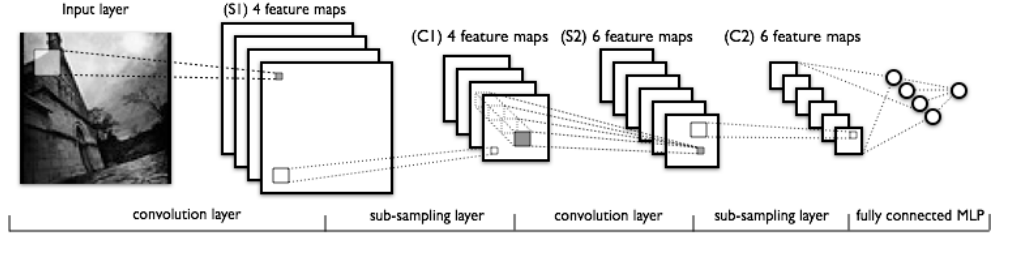2.代码总体架构（手绘）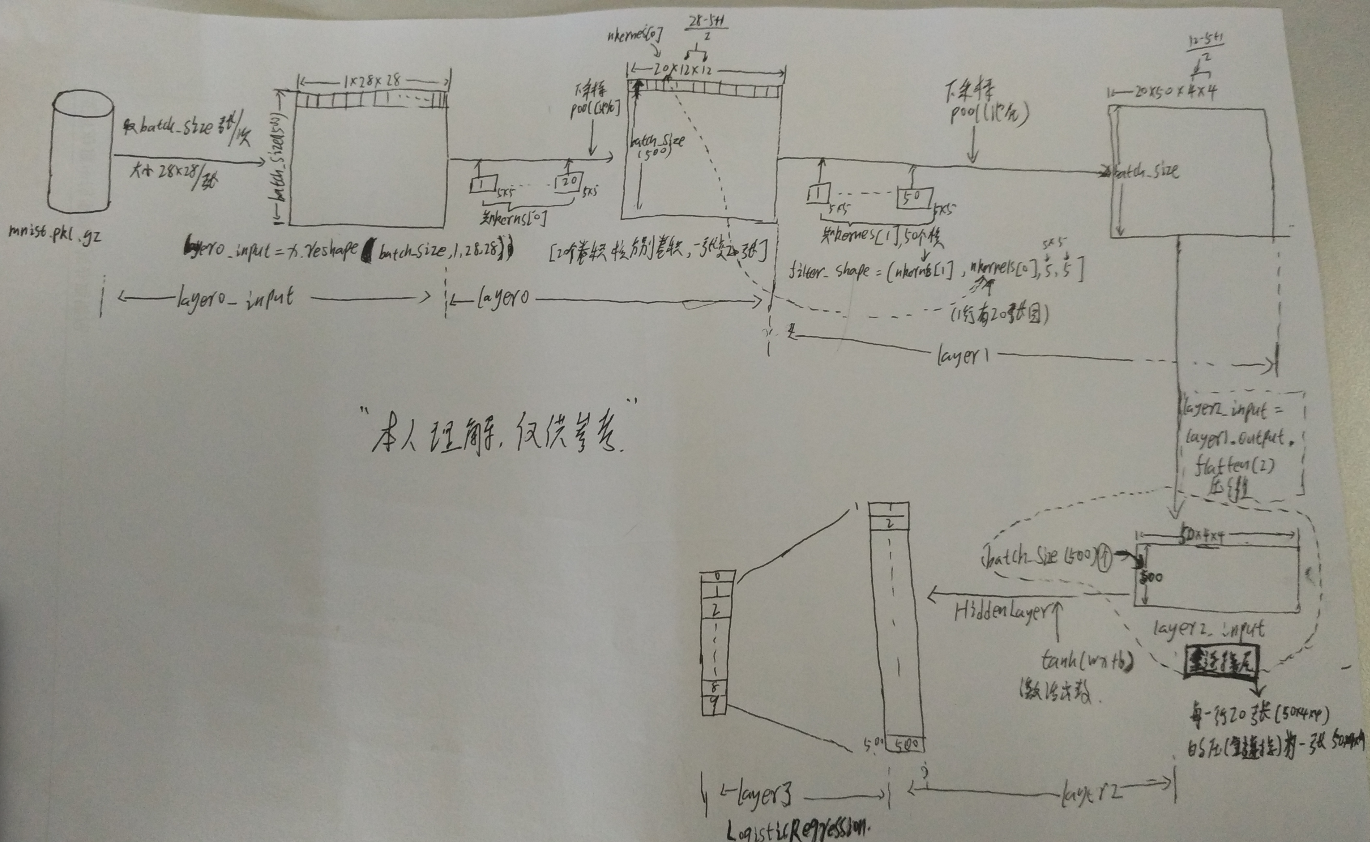3.代码分析
#【LeNet网络总框架】：LeNetConvPoolLayer.py 看的时候结合mlp.py

<pre name="code" class="python"># -*- coding: utf-8 -*-
#来自网络
import os
import sys
import timeit
import pp
import numpy
import theano
import theano.tensor as T
from theano.tensor.signal import downsample
from theano.tensor.nnet import conv
from mlp import HiddenLayer,LR as LogisticRegression,load_data

#先从main函数： evaluate_lenet5()看起
#该函数实现了卷积+采样（池化）的过程
class LeNetConvPoolLayer(object):
"""卷积层+采样层"""
#输入的参数
def __init__(self, rng, input, filter_shape, image_shape, poolsize=(2, 2)):
"""
rng,input。
filter_shape: 长度为4的元组或list
filter_shape: (过滤器数目, 输入特征图数目, 过滤器高度, 过滤器宽度)

image_shape: 长度为4的元组或list
image_shape: (样本块大小, 输入特征图数目, 图像高度, 图像宽度)

poolsize: 长度为2的元组或list
poolsize: 下采样的shape大小(#rows, #cols)
"""

# 断言，确定image_shape的1号元素与filter_shape的1号元素相等 如不成立会引发一个错误
# 因为从以上定义中可以知道，这个元素代表输入特征图的数量
assert image_shape == filter_shape
self.input = input

# prod()返回元素之积。如果filter_shape=(2,4,3,3),
# 那么filter_shape[1:]=(4,3,3)
# prod(filter_shape[1:])=4*3*3=36
#（这里试根据tutorial来的）
fan_in = numpy.prod(filter_shape[1:])
# "num output feature maps * filter height * filter width" /
#   pooling size
fan_out = (filter_shape * numpy.prod(filter_shape[2:]) /
numpy.prod(poolsize))

# 用随机均匀分布初始化权值W
W_bound = numpy.sqrt(6. / (fan_in + fan_out))
#theano.shared 就是一个共享变量，在程序的任何地方都可以用，而且值相同theano.shared（值，类型）
#numpy.asarray 将列表转化为数组
#numpy.random.RandomState.uniform 统一随机生成数据的范围（）
'''
Draw samples from the distribution:
>>>

>>> s = np.random.uniform(-1,0,1000)

All values are within the given interval:
>>>

>>> np.all(s >= -1)
True
>>> np.all(s < 0)
True

'''
self.W = theano.shared(
numpy.asarray(
rng.uniform(low=-W_bound, high=W_bound, size=filter_shape),
dtype=theano.config.floatX
),
borrow=True
)

# 每一张输出特征图都有一个一维的偏置值，初始化为0。偏置也是属于共享变量的
b_values = numpy.zeros((filter_shape,), dtype=theano.config.floatX)
self.b = theano.shared(value=b_values, borrow=True)

# 将输入特征图与过滤器进行卷积操作
conv_out = conv.conv2d(
input=input,
filters=self.W,
filter_shape=filter_shape,
image_shape=image_shape
)

# 用maxpooling方法下采样每一个张特征图 池化
pooled_out = downsample.max_pool_2d(
input=conv_out,
ds=poolsize,
ignore_border=True
)

# 先把偏置进行张量扩张，由1维扩展为4维张量(1*2*1*1)
# 再把扩展后的偏置累加到采样输出
# 把累加结果送入tanh非线性函数得到本层的网络输出
self.output = T.tanh(pooled_out + self.b.dimshuffle('x', 0, 'x', 'x'))

# store parameters of this layer
self.params = [self.W, self.b]

# keep track of model input
self.input = input

#原始参数：learning_rate：学习速率 n_epochs：就是整个数据集 mnist.pkl.gz中全部重复学习次数
#卷积核的个数：nkerns=[20, 50]【nkerns是layer1中卷积核个数，同理nkernes为layer2】
#每一次学习输入的数据：batch_size=500张 后面会计算出 n_train_batches 为100次：500*100=50000张训练图
def evaluate_lenet5(learning_rate=0.1, n_epochs=1000,
dataset='mnist.pkl.gz',
nkerns=[20, 50], batch_size=1000):
""" Demonstrates lenet on MNIST dataset
实验数据集是MNIST数据集。
:type learning_rate: float
:param learning_rate: learning rate used (factor for the stochastic

:type n_epochs: int
:param n_epochs: maximal number of epochs to run the optimizer
n_epochs是最大迭代次数。一次完整迭代包括计算完所有完整数据，即(总数size/batch_size)次

:type dataset: string
:param dataset: path to the dataset used for training /testing (MNIST here)
数据集路径

:type nkerns: list of ints
:param nkerns: number of kernels on each layer
卷积核数目。第一个下采样层有20个卷积核，第二个下采样有50个卷积核。
一个卷积核经过卷积计算会生成一张特征图。
(我认为卷积核就相当于神经元的个数，对应着权值的元素个数)
"""
rng = numpy.random.RandomState(23455)#产生随机数

train_set_x, train_set_y = datasets#训练集
valid_set_x, valid_set_y = datasets#验证集
test_set_x, test_set_y = datasets#测试集

# compute number of minibatches （计算需要多少次进行） for training, validation and testing
#得到各训练集中的总数
n_train_batches = train_set_x.get_value(borrow=True).shape
n_valid_batches = valid_set_x.get_value(borrow=True).shape
n_test_batches = test_set_x.get_value(borrow=True).shape
#计算以batch_size为一批计算需要多少次迭代
n_train_batches /= batch_size
n_valid_batches /= batch_size
n_test_batches /= batch_size

# allocate symbolic variables for the data
#这里的index也是个变量，后面的minibatch_index会对其赋值，表示选择第几批数据，每批batch_size个图像
index = T.lscalar()
#这里的x,y就是符号象征，相当于定义变量，后面的层中会有对应的gives[x：  y： ]与之对应赋值
# start-snippet-1
x = T.matrix('x')   # the data is presented as rasterized images
y = T.ivector('y')  # the labels are presented as 1D vector of
# [int] labels

######################
# BUILD ACTUAL MODEL #
######################
print '... building the model'

# 构造第0层的输入数据。就是把形状shape为(batch_size,28*28)数据块转化为四维(batch_size,1,28,28)
# (batch_size,1，28*28)就是有batch_size行，一行对应一个样本，每行有28*28列，是对应样本的具体数据【就是构造一个大矩阵，每行放1个图，大小为28*28，放batch_size行】
#就是把刚才的x弄成 batch_size[行]*（1个×(28*28)）【列】大小（不理解见手绘图）
layer0_input = x.reshape((batch_size, 1, 28, 28))

# Construct the first convolutional pooling layer:（具体见cnn卷积、池化后图像尺寸的变化）
# filtering reduces the image size to (28-5+1 , 28-5+1) = (24, 24)
# maxpooling reduces this further to (24/2, 24/2) = (12, 12)
# 4D output tensor is thus of shape (batch_size, nkerns, 12, 12)
layer0 = LeNetConvPoolLayer(
rng,
input=layer0_input,
image_shape=(batch_size, 1, 28, 28),
#一次输入batch_size张图，每张分别与一个卷积模板进行卷积（这里面的1指的是一行有1个（28*28）的图像）
filter_shape=(nkerns, 1, 5, 5),#给出这个模板的大小 nkernes 50个 5*5 的模板
#每张和50张模板卷积，大小为图像大小为28*28 也即输入的数据大小为batch_size[行]*1×(28*28)【列】大小
poolsize=(2, 2)#给出池化的大小
)

# Construct the second convolutional pooling layer
# filtering reduces the image size to (12-5+1, 12-5+1) = (8, 8)
# maxpooling reduces this further to (8/2, 8/2) = (4, 4)
# 4D output tensor is thus of shape (batch_size, nkerns, 4, 4)
layer1 = LeNetConvPoolLayer(
rng,
input=layer0.output,
image_shape=(batch_size, nkerns, 12, 12),
#第二层的输入batch_size×nkerns张图片,每张大小为12*12 就是输入数据的大小为 batch_size【行】×（nkerns*(12*12)）【列】
#or (500, 50 * 12 * 12) = (500, 7200)
filter_shape=(nkerns, nkerns, 5, 5),
#卷积核为20(nkerns)×50（nkerns）个每个大小为5*5类比上句
poolsize=(2, 2)
)

# the HiddenLayer being fully-connected, it operates on 2D matrices of
# shape (batch_size, num_pixels) (i.e matrix of rasterized images).
# This will generate a matrix of shape (batch_size, nkerns * 4 * 4),
# or (500, 50 * 4 * 4) = (500, 800) with the default values.
layer2_input = layer1.output.flatten(2)

# construct a fully-connected sigmoidal layer in mlp.py  隐层，与输入层是全连接
layer2 = HiddenLayer(
rng,
input=layer2_input,
n_in=nkerns * 4 * 4,#输入数据的大小 20×4*4=320
n_out=500,#最终输出500个向量
activation=T.tanh#非线性激活函数
)

# 就是mlp.py 中的LR函数 逻辑回归 在该层中权重W以及偏置b均初始化为0（注意只是在该层中的初始化），并设置了回归函数
layer3 = LogisticRegression(input=layer2.output, n_in=500, n_out=10)

# the cost we minimize during training is the NLL of the model
cost = layer3.negative_log_likelihood(y)#执行代价函数
#测试集函数
test_model = theano.function(
[index],#批次索引
layer3.errors(y),#调用时执行这个计算错误的函数【测试数据集上的错误】
givens={#输入对应的数据以及标签
x: test_set_x[index * batch_size: (index + 1) * batch_size],
y: test_set_y[index * batch_size: (index + 1) * batch_size]
}
)
#验证集函数
validate_model = theano.function(
[index],
layer3.errors(y),#同理计算验证集数据错误
givens={
x: valid_set_x[index * batch_size: (index + 1) * batch_size],
y: valid_set_y[index * batch_size: (index + 1) * batch_size]
}
)

# create a list of all model parameters to be fit by gradient descent（梯度下降）
params = layer3.params + layer2.params + layer1.params + layer0.params

# create a list of gradients （梯度）for all model parameters

# train_model is a function that updates the model parameters by
# SGD Since this model has many parameters, it would be tedious to
# manually create an update rule for each model parameter. We thus
# create the updates list by automatically looping over all
(param_i, param_i - learning_rate * grad_i)
]
#训练函数
train_model = theano.function(
[index],
cost,
givens={
x: train_set_x[index * batch_size: (index + 1) * batch_size],
y: train_set_y[index * batch_size: (index + 1) * batch_size]
}
)
# end-snippet-1

###############
# TRAIN MODEL #
###############
print '... training'
# early-stopping parameters
patience = 10000  # look as this many examples regardless
patience_increase = 2  # wait this much longer when a new best is 如果训练的好的话加倍训练次数

#当新的验证误差是原来的0.995倍时，才会更新best_validation_loss。即误差小了，但是至少要小了0.99
improvement_threshold = 0.995  # a relative improvement of this much is
# considered significant
validation_frequency = min(n_train_batches, patience / 2)#以最小的训练次数去验证
# go through this many
# minibatche before checking the network
# on the validation set; in this case we
# check every epoch

best_validation_loss = numpy.inf
best_iter = 0#最好得分对应的训练次数
test_score = 0.
start_time = timeit.default_timer()

epoch = 0
done_looping = False
#n_epochs:整个'mnist.pkl.gz'跑几遍
while (epoch < n_epochs) and (not done_looping):
epoch = epoch + 1
for minibatch_index in xrange(n_train_batches):

iter = (epoch - 1) * n_train_batches + minibatch_index#现在一共训练的数量

if iter % 100 == 0: #每隔100次打印一次
print 'training @ iter = ', iter
cost_ij = train_model(minibatch_index)#真正的调用这个函数去训练（从高层调用激活所有的层）
#print 'minibatch_index=',minibatch_index
#validation_frequency = min(n_train_batches, patience / 2) 每隔validation_frequency进行一次验证
if (iter + 1) % validation_frequency == 0:

# compute zero-one loss on validation set 这里调用验证模型去计算layer3.errors
validation_losses = [validate_model(i) for i
in xrange(n_valid_batches)]
this_validation_loss = numpy.mean(validation_losses)#计算均值
print('epoch %i, minibatch %i/%i, validation error %f %%' %
(epoch, minibatch_index + 1, n_train_batches,
this_validation_loss * 100.))

# if we got the best validation score until now
if this_validation_loss < best_validation_loss:

#improve patience if loss improvement is good enough
if this_validation_loss < best_validation_loss *  \
improvement_threshold:
# validation_frequency = min(n_train_batches, patience / 2) 会影响到验证集验证频率
patience = max(patience, iter * patience_increase)

# save best validation score and iteration number 更新最好的记录
best_validation_loss = this_validation_loss
best_iter = iter

# test it on the test set 在测试集上测试
test_losses = [
test_model(i)
for i in xrange(n_test_batches)
]
test_score = numpy.mean(test_losses)
print(('     epoch %i, minibatch %i/%i, test error of '
'best model %f %%') %
(epoch, minibatch_index + 1, n_train_batches,
test_score * 100.))

if patience <= iter:
done_looping = True
break

end_time = timeit.default_timer()
print('Optimization complete.')
print('Best validation score of %f % evaluate_lenet5()% obtained at iteration %i, '
'with test performance %f %%' %
(best_validation_loss * 100., best_iter + 1, test_score * 100.))
print >> sys.stderr, ('The code for file ' +
os.path.split(__file__) +
' ran for %.2fm' % ((end_time - start_time) / 60.))

if __name__ == '__main__':
evaluate_lenet5()

def experiment(state, channel):
evaluate_lenet5(state.learning_rate, dataset=state.dataset)

#【具体细节实现：结合mlp.py】

展开全文深度学习 theano
• 基于Tensorflow实现Lenet 5 简单回顾一下Lenet 5的基本结构 在此之前导入相关模块，以下的代码均基于此来运行 import tensorflow as tf from tensorflow . examples . tutorials . mnist import input...
看了卷积神经网络（CNN）的原理及介绍，想着自己动手解决一个案例，在网上也看了很多博客，这里整理一下，顺便记录一下自己解决一个完成的CNN实例的过程，以便以后方便看。
如果有不足之处，欢迎大家指正。
数据获取与可视化操作
1、下载minst数据集
在网上可以很容易找到minst数据集，这里为了方便大家，直接给出链接，大家直接点击下载即可.。注意下载后不要解压缩。
2、数据的读取
利用python的pickle模块以及gzip库直接读取文件
import pickle
import gzip
# 数据的读取
f = gzip.open("D:\\wangyang\\图像识别\\程序\\CNN\\minst.gz", "rb")
train_set, valid_set, test_set = pickle.load(f, encoding="bytes")
#注意后面的编码参数encoding不能省略，否则会报错
f.close()
#记得及时关闭文件

到这里数据的读取工作就已经完成了，值得注意的是训练集train_set是一个元组（tuple，一共有两个元素），第一个元素是一个多维数组（np.ndarray），存储了50000个28*28的二维数组（可以看成是一个矩阵），也就是说mnist.pkl.gz库中图像的大小是28 * 28的。
3.数据的可视化（tile）
为了更清楚看到数据究竟是什么样子的，我们需要对数据进行可视化操作（当然这不是必须的）对训练集数据进行可视化操作，结果如下图所示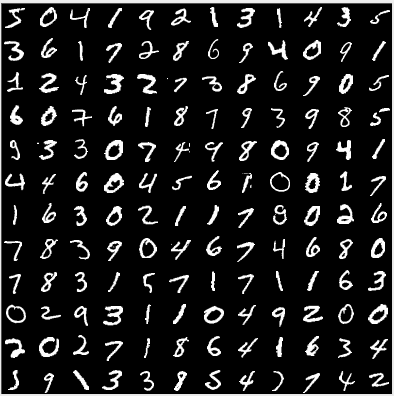具体程序如下所示
class DataofVisualization(object):
"""对数据的可视化操作，将数据tile出来"""

def normalize(self, data):
"""
对数据进行归一化操作，这里采用线性归一化方法——Min-Max归一化
X_norm=(X-min)/(X_max-X_min)
"""
from sklearn import preprocessing
X = preprocessing.MinMaxScaler()
return X.fit_transform(data)

def tile_raster_images(self, X, image_shape, tile_shape, tile_spacing=(0, 0), normalize_rows=True, output_pixel_vals=True):
"""
把图片按贴砖的形式打印出来
image_shape:每一块砖的高和宽；
tile_shape:在横纵方向上分别有多少砖；
tile_spacing:砖与砖之间的距离
normalize_rows:是否对砖进行归一化；
output_pixel_vals:是否对砖以图像的形式进行显示
"""
# 对参数进行断言（asser），确保它们都是二维数组，否则报错
assert len(image_shape) == 2
assert len(tile_shape) == 2
assert len(tile_spacing) == 2

output_shape = [(ishp + tsp) * tshp - tsp for ishp, tshp, tsp in zip(image_shape,
tile_shape, tile_spacing)]
# 计算最后的"大砖"高和宽

im_H, im_w = image_shape
Hs, Ws = tile_spacing
dt = "uint8" if output_pixel_vals else X.dtype  # 确定数据的编码格式
output_array = np.zeros(output_shape, dtype=dt)

# 开始贴砖
for i in range(tile_shape):
for j in range(tile_shape):
if i * tile_shape + j < X.shape:
this_x = X[i * tile_shape + j]
this_image = self.normalize(this_x.reshape(
image_shape)) if normalize_rows else this_x.reshape(image_shape)

c = 255 if output_pixel_vals else 1
output_array[i * (im_H + Hs):i * (im_H + Hs) + im_H, j * (im_w + Ws):
j * (im_w + Ws) + im_w] = this_image * c
return output_array
mydata = DataofVisualization()
X = train_set
arr = mydata.tile_raster_images(X, image_shape=(28, 28),
tile_shape=(12, 12), tile_spacing=(5, 5))
img = Image.fromarray(arr)
img.show()


归一化方法有两种形式，一种是把数变为（0，1）之间的小数，一种是把有量纲表达式变为无量纲表达式。主要是为了数据处理方便提出来的，把数据映射到0～1范围之内处理，更加便捷快速，应该归到数字信号处理范畴之内

注意上面的利用到了归一化处理，为了简单起见只用了线性归一化方法，对其他归一化方法感兴趣的同学可以看这篇博客。
卷积神经网络
关于卷积神经网络网上已经有很多优秀的博客了，这里就不再多说什么了，感兴趣的可以看看下面这篇博客
卷积神经网络详解 - 卷积层逻辑篇
接下来我想复现经典的卷积神经网络——Lenet-5模型。
前期准备工作，请确保以下模块以及安装成功
基于theano实现Lenet 5
import os
import sys
import timeit
import theano
# theano与tensorfloe类似，都是基于建立神经网络的组件
import theano.tensor as T
from theano.tensor.signal import pool
from theano.tensor.nnet import conv
from mlp import HidderLayer

期间会出现如下问题
Q：No module named ‘logistic_sgd’
A：对照着博客一步一步解决就好了（注意最好把文件放在你当前工程的目录下）
Q:出现下列问题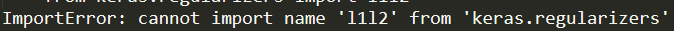将keras版本退化到1.2.2版本，win+r调出命令窗口，输入如下命令
pip install keras == 1.2.2

Q：缺少模块
A：根据错误信息，利用pip install下载缺少的模块即可
Q：出现下列问题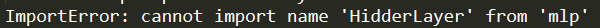A：后来发现这个目前mlp类中已经不支持HidderLayer了，在网上找了很久都没有找到对应的解决办法，希望以后有大佬能够解决吧
到这里我实在是不知道怎么看下去了，本来就是初学者，哈哈哈哈，所以改用基于Tensorflow来实现
基于Tensorflow实现Lenet 5
简单回顾一下Lenet 5的基本结构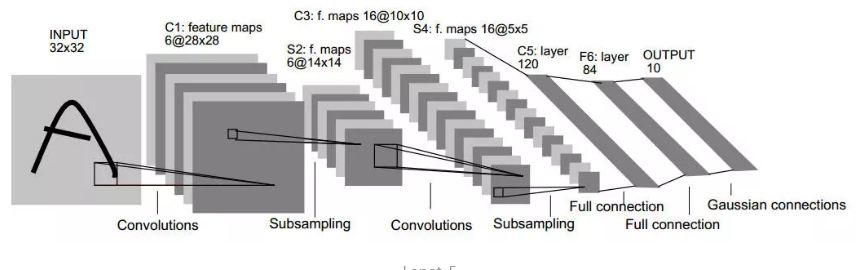在此之前导入相关模块，以下的代码均基于此来运行
import tensorflow as tf
from tensorflow.examples.tutorials.mnist import input_data

运行编译一下，观察是否出现错误，如果报错根据错误信息对应解决
（一般多是没有对应的模块，win+r调出命令窗口利用pip命令下载对应的模块即可）
因为笔者本身就是一个新手，所有下面的东西难免会出错，同时也会有些啰嗦，还请大家多多担待。
数据的读取
同样的，要实现一个卷积神经网络最开始的就是数据的读取工作，所以接下来实现数据的读取操作
mnist = input_data.read_data_sets(
'D:\\wangyang\\图像识别\\程序\\CNN\\mnist.gz', one_hot=True)

sess = tf.InteractiveSession() #创建一个对话

运行上述代码之后会发现之前的文件夹中多了一个文件夹，里面是这样的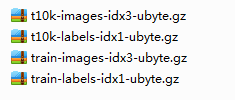是不是很神奇？其实不是，秘密就在那一行命令中。
首先来说明一下input_data是手写数字识别的一个模块，其中read_data_sets方法的具体含义不再多说，相关参数如下
def read_data_sets(train_dir,
fake_data=False,
one_hot=False,
dtype=dtypes.float32,
reshape=True,
validation_size=5000,
seed=None,
source_url=DEFAULT_SOURCE_URL)

我们一般常用第一个参数（数据集的绝对路径，即在电脑中的位置）和第三个参数，直白点说就是用0或1去描述图片上的数字，只能有一个1，它在哪一位就代表数字几，其他位均为0。最后关于InteractiveSession就不再多说了，感兴趣的自己点击看看。（TensorFlow中的变量是惰性加载的，如果你想看到变量的值，必须建立一个对话（Session））
训练数据
# 训练数据
x = tf.placeholder("float", shape=[None, 784])
# 训练标签数据
y_ = tf.placeholder("float", shape=[None, 10])
# 把x更改为4维张量，第1维代表样本数量，第2维和第3维代表图像长宽， 第4维代表图像通道数, 1表示黑白
x_image = tf.reshape(x, [-1, 28, 28, 1])
#因为minst数据集中图像的大小都是28*28的，所以第二个参数和第三个参数均为28，第一个参数为-1表示大小不确定

其中tf.placeholder的用法如下，只有dtype参数是必选参数，其余均是可选参数。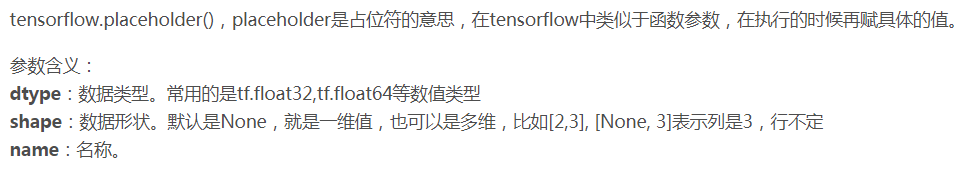卷积层C1

# 第一层：卷积层
# 过滤器大小为5*5, 当前层深度为1， 所以过滤器的深度为1，一共6个过滤器，即6个卷积核
conv1_weights = tf.get_variable("conv1_weights", [5, 5, 1, 6], initializer=tf.truncated_normal_initializer(stddev=0.1))#过滤器，即卷积核
#偏置项，因为有6个卷积核，所以需要6个偏置项
conv1_biases = tf.get_variable("conv1_biases", , initializer=tf.constant_initializer(0.0))
# 移动步长为1, 不使用全0填充
conv1 = tf.nn.conv2d(x_image, conv1_weights, strides=[1, 1, 1, 1], padding='VALID')
# 激活函数Relu去线性化
#relu1即为本层的输出，亦为下一层的输入

代码解释
注意：get_variable是创建一个变量并初始化，第一个参数的变量名，第二个参数是大小，第三个参数用于初始化，详细说明点击此处并且两个初始化方法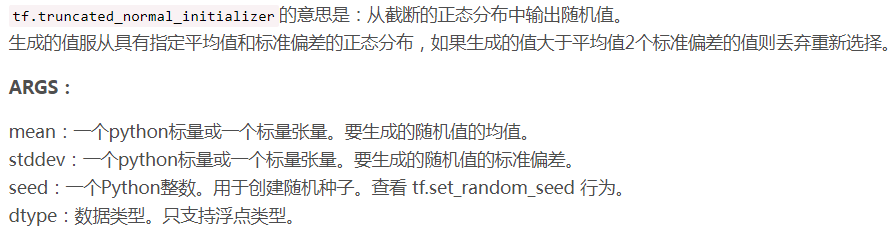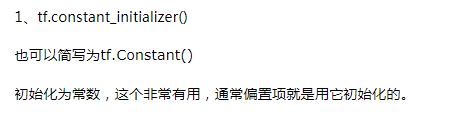conv1 = tf.nn.conv2d(x_image, conv1_weights, strides=[1, 1, 1, 1], padding='VALID')

中strides参数，第一个和第四个是1，不能改变，第二个和第三个分别指水平滑动和垂直滑动的步长值（一般来说是一样的，这里就是说步长为1），参数padding通俗的说就是决定是否补0，后面是padding='VALID'就是不进行补0操作的，详细解析点击此处
这句话的命令实际就是在进行卷积运算，tf.nn.conv2d的具体用法点击此处，这里就不再多说了，只要明白这一步是在进行卷积运算就好了。
relu1 = tf.nn.relu(tf.nn.bias_add(conv1, conv1_biases))

函数tf.nn.relu就是我们通常立即的Relu激活函数（相关用法），即函数tf.nn.bias_add表示加上偏置项，用一维向量加到矩阵的每一行上去。
到这里就已经结束了第一层卷积。
逻辑分析
输入：28 * 28大小的矩阵，深度为1，数目未知
卷积核：5 * 5，深度为1，一共6个
偏置项：constant，一维，一共6个
步长S：S=1
输出：6个特征图，每一个大小24*24，深度为1（与输入矩阵的大小保持一致）
因为计算公式如下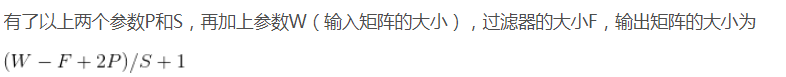池化层S2
# 第二层：最大池化层
# 池化层过滤器的大小为2*2, 移动步长为2，不使用全0填充
pool1 = tf.nn.max_pool(relu1, ksize=[1, 2, 2, 1], strides=[

代码解释
这里采用的最大池化，在池化层中一般使用非重叠池化，所以这里步长设置为了2，池化大小为（2,2），这里通过tf.nn.max_pool函数来实现了池化层的功能，相关用法请点击此处
逻辑分析
输入：24 * 24大小的矩阵，一个有6个（也就是特征图）
池化大小：2 * 2
步长（S）：S=2
输出：12 * 12大小的特征图，一共有6个（池化不会改变特征图的个数，只会改变每个特征图的大小）
卷积层C3
# 第三层：卷积层
conv2_weights = tf.get_variable("conv2_weights", [5, 5, 6, 16], initializer=tf.truncated_normal_initializer(
stddev=0.1))  # 过滤器大小为5*5, 当前层有6个输入， 过滤器的个数为16
conv2_biases = tf.get_variable(
"conv2_biases", , initializer=tf.constant_initializer(0.0))
conv2 = tf.nn.conv2d(pool1, conv2_weights, strides=[
1, 1, 1, 1], padding='VALID')  # 移动步长为1, 使用全0填充

代码解释
相关命令与C1层类似（只是相关的参数不一样），这里就不再重复了。这里仅说明
conv2_weights = tf.get_variable("conv2_weights", [5, 5, 6, 16], initializer=tf.truncated_normal_initializer(
stddev=0.1))

中的第二个参数中的第三个为什么是6，因为该层的输入就是池化层S2的输出，而S2的输出一共有6个特征图，所以这里为6
逻辑分析
输入：12 * 12的特征图，一共6个，每个深度为1
卷积核：5 * 5的矩阵，一共16个，每个深度也为1
步长（S）：S=1
输出：8 * 8的特征图，得到16个特征图(一般是几个卷积核就会得到几个特征图)
值得注意的是：
卷积层S3与S1是有不同的，最主要的区别就是连接方式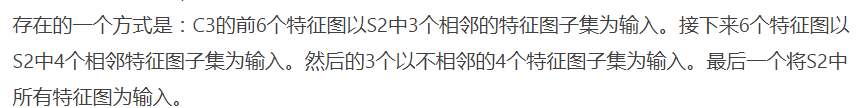输入的6个特征图与卷积层中16个卷积核的连接方式如下图所示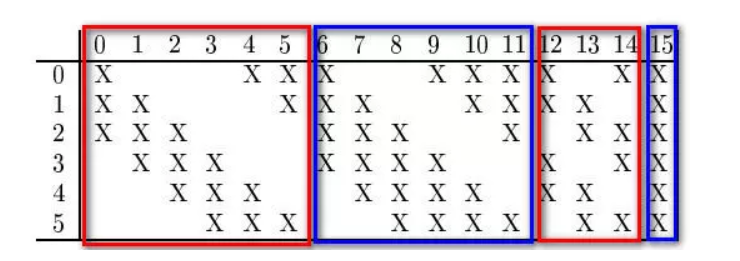简单的就是
C2得到了6个特征图，S3中有16个卷积核，最后经过卷积层S3，得到了16个特征图
1、前6个特征图是以C2中的三个特征图作为输入，作用到6个卷积核中，得到了6个
eg:用特征图0,1,2作为输入，作用到卷积核0中，得到了一个特征图；用特征图1,2,3作用到卷积核1中又得到一个特征图（也就是第一个红色的方框里的形式），以此类推可以得到6个特征图
2、接下来的6个特征图是用连续4个特征图作为输入，作用到另外6个卷积核，又可以得到6个特征图
eg:用特征图0,1,2,3作为输入，作用到卷积核6中，得到一个特征图；用特征图1,2,3,4作为输入，作用到卷积核7中又可以得到一个特征图（即第二个蓝色方框中的形式），以此类推可以得到6个特征图
3、接下来的3个特征图是用间隔的4个特征图作用到3个卷积核中得到的
eg:用特征图0,1,3,4作为输入，作用到卷积核12中，得到一个特征图；用特征图1，2,4,5作为输入，又可以得到一个特征图（即第三个红色方框中的形式），以此类推得到3个特征图
4、最后一个特征图是用6个特征图作为输入，作用到最后一个卷积核得到的
eg:用特征图0,1,2,3,4,5作为输入，作用到卷积核15中得到第16个特征图
池化层C4
# 第四层：最大池化层
# 池化层过滤器的大小为2*2, 移动步长为2，使用全0填充
pool2 = tf.nn.max_pool(relu2, ksize=[1, 2, 2, 1], strides=[

代码解释
这一层代码池化层C2类似，这里就不在重复了
逻辑分析
输入：8*8的特征图，一共有16个
池化器：2 * 2
步长：S=2
输出：4 * 4的特征图，一共16个
全连接层C5
# 第五层：全连接层
fc1_weights = tf.get_variable("fc1_weights", [
4 * 4 * 16, 120], initializer=tf.truncated_normal_initializer(stddev=0.1))  # 7*7*64=3136把前一层的输出变成特征向量
fc1_baises = tf.get_variable(
"fc1_baises", , initializer=tf.constant_initializer(0.1))
pool2_vector = tf.reshape(pool2, [-1, 4 *4* 16])
fc1 = tf.nn.relu(tf.matmul(pool2_vector, fc1_weights) + fc1_baises)

# 为了减少过拟合，加入Dropout层
keep_prob = tf.placeholder(tf.float32)
fc1_dropout = tf.nn.dropout(fc1, keep_prob)

代码解释
这一层在Lenet论文中也是卷积层，只不过在经过卷积核作用以后它变成了一个1*1的，起到了一个类似全连接的作用
fc1_weights = tf.get_variable("fc1_weights", [
4 * 4 * 16, 120], initializer=tf.truncated_normal_initializer(stddev=0.1))

创建一个张量，因为池化层的输入是4 * 4的，一共有16个，经过这一层后要得到120个1 * 1的特征图，所以要创建一个[4*4*16,120]的张量
fc1 = tf.nn.relu(tf.matmul(pool2_vector, fc1_weights) + fc1_baises)

函数tf.matmul表示两个矩阵相乘，具体用法点击此处
fc1_dropout = tf.nn.dropout(fc1, keep_prob)

函数tf.nn.dropout()是tensorflow里面为了防止或减轻过拟合而使用的函数，它一般用在全连接层，具体用法点击此处
逻辑分析
输入：4 * 4的特征图，一共16个
输出：1 * 1的特征图，一个120个
全连接层F6
# 第六层：全连接层
fc2_weights = tf.get_variable("fc2_weights", [
120, 10], initializer=tf.truncated_normal_initializer(stddev=0.1))  # 神经元节点数120, 分类节点10，也就是输入为120，输出为10
fc2_biases = tf.get_variable(
"fc2_biases", , initializer=tf.constant_initializer(0.1))
fc2 = tf.matmul(fc1_dropout, fc2_weights) + fc2_biases


代码解释
与上一层大致一样，最大的区别是上一层虽然叫做全连接层，但是本质还是一个卷积层，所以需要通过激活函数，这一层就是完完全全的全连接层，所以不需要激活函数
逻辑分析
输入：神经节点120个
输出：分类节点10个
输出层Output
# 第七层：输出层
# softmax
y_conv = tf.nn.softmax(fc2)
# 定义交叉熵损失函数
cross_entropy = tf.reduce_mean(-tf.reduce_sum(y_ *
tf.log(y_conv), reduction_indices=))

# 选择优化器，并让优化器最小化损失函数/收敛, 反向传播

# tf.argmax()返回的是某一维度上其数据最大所在的索引值，在这里即代表预测值和真实值
# 判断预测值y和真实值y_中最大数的索引是否一致，y_conv的值为1-10概率
correct_prediction = tf.equal(tf.argmax(y_conv, 1), tf.argmax(y_, 1))

# 用平均值来统计测试准确率
accuracy = tf.reduce_mean(tf.cast(correct_prediction, tf.float32))

代码解释
函数tf.nn.softmax主要是将N * 1的向量转成N * 1的向量（每个元素的大小在0-1之间，也就是属于某一类的概率），具体用法点击此处。
cross_entropy = tf.reduce_mean(-tf.reduce_sum(y_ *
tf.log(y_conv), reduction_indices=))

函数tf.log用来求自然对数（底数为e），具体用法点击此处
函数tf.reduce_sum是求和，该命令的意思是对矩阵y*tf.log(y_cov)横向求和，具体用法点击此处
函数tf.reduce_mean是用来求平均值，具体用法点击此处
另外交叉熵损失函数如下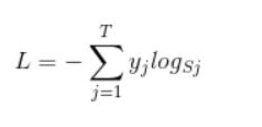train_step = tf.train.AdamOptimizer(1e-4).minimize(cross_entropy)

correct_prediction = tf.equal(tf.argmax(y_conv, 1), tf.argmax(y_, 1))

函数tf.argmax表示返回某一维度上最大值所在的索引值（该命令是返回某一行最大值所在的位置，因为y_conv和y_是一个行向量），详细用法点击此处
函数tf.equal用来判断两个张量是否相等（逐个元素进行比较），具体用法点击此处
# 用平均值来统计测试准确率
accuracy = tf.reduce_mean(tf.cast(correct_prediction, tf.float32))

函数tf.cast进行张量的数据类型转换，将布尔值转成浮点数（ture变成1，false变成0），具体用法点击此处
逻辑分析
输入：1*10的向量
输出：1*10的向量，但是向量中的值均在0-1之间
训练数据
# 开始训练
sess.run(tf.global_variables_initializer())
for i in range(5000):#这里仅训练五千次
batch = mnist.train.next_batch(100)#批量读取数据
if i % 100 == 0:
train_accuracy = accuracy.eval(
feed_dict={x: batch, y_: batch, keep_prob: 1.0})  # 评估阶段不使用Dropout
print("step %d, training accuracy %g" % (i, train_accuracy))
# 训练阶段使用50%的Dropout
train_step.run(feed_dict={x: batch, y_: batch, keep_prob: 0.5})
# 在测试数据上测试准确率
print("test accuracy %g" % accuracy.eval(
feed_dict={x: mnist.test.images, y_: mnist.test.labels, keep_prob: 1.0}))

代码解释
函数feed_dict是用来给之前占位符赋值，即把batch给x等。
运行结果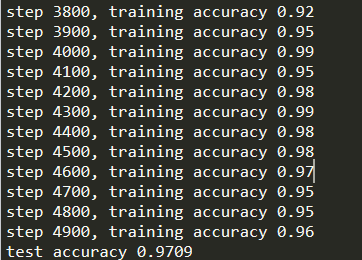完整代码
完整的代码请点击此处下载
参考博客
数据集可视化——tile（“贴砖”）
深度学习 CNN卷积神经网络 LeNet-5详解


展开全文图像识别
• 本文先分析LeNet-5模型的结构，然后基于LeNet-5模型写了TensorFlow代码实现mnist数字识别，代码部分进行了详细注解，目前也在学习阶段，有错误欢迎指出，大家一起学习。 LeNet-5模型结构图如下图： LeNet-5模型...
       本文先分析了LeNet-5模型的结构，然后基于LeNet-5模型写了TensorFlow代码实现mnist数字识别，代码部分进行了详细注解，目前也在学习阶段，有错误欢迎指出，大家一起学习。
LeNet-5模型结构图如下图：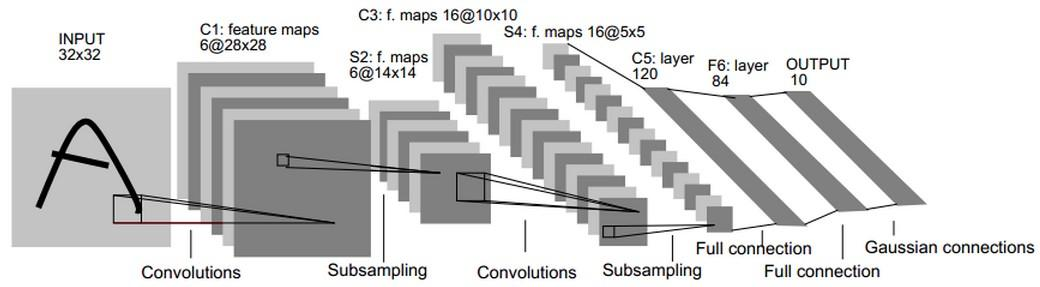LeNet-5模型总共有7层。
第一层：卷积层
第一层卷积层的输入为原始的图像，原始图像的尺寸为32×32×1。卷积层的过滤器尺寸为5×5，深度为6，不使用全0补充，步长为1。由于没有使用全0补充，所以这一层的输出的尺寸为32-5+1=28，深度为6。这一个卷积层总共有5×5×1×6+6=156个参数，其中6为偏置项参数个数，卷积层的参数个数只和过滤器的尺寸，深度以及当前层节点矩阵的深度有关。因为下一层节点矩阵有28×28×6=4704个节点，每个节点和5×5=25个当前层节点相连，所以本层卷积层总共有4704×(25+1)=122304个连接。
第二层：池化层
这一层的输入为第一层的输出，是一个28×28×6的节点矩阵。本层采用的过滤器大小为2×2，步长为2，所以本层的输出矩阵大小为14×14×6。
第三层：卷积层
本层的输入矩阵大小为14×14×6，采用的过滤器大小为5×5，深度为16，不使用全0补充，步长为1。这一层的输出的尺寸为14-5+1=10，深度为16，即输出矩阵大小为10×10×16。本层参数有5×5×6×16+16=2416个，连接有10×10×16×(5×5+1)=41600个。
第四层：池化层
本层的输入矩阵大小为10×10×16，采用的过滤器大小为2×2，步长为2，本层的输出矩阵大小为5×5×16。
第五层：全连接层
本层的输入矩阵大小为5×5×16，在LeNet-5模型的论文中将这一层称为卷积层，但是因为过滤器的大小就是5×5，所以和全连接层没有区别，这里直接看成全连接层。本层输入为5×5×16矩阵，将其拉直为一个长度为5×5×16的向量，即将一个三维矩阵拉直到一维空间以向量的形式表示，这样才可以进入全连接层进行训练。本层的输出节点个数为120，所以总共有5×5×16×120+120=48120个参数。
第六层：全连接层
本层的输入节点个数为120个，输出节点个数为84个，总共有120×84+84=10164个参数。
第七层：全连接层
本层的输入节点个数为84个，输出节点个数为10个，总共有84×10+10=850个参数。
接下来以TensorFlow代码展示一个基于LeNet-5模型的mnist数字识别代码。
mnist数据集png图片格式下载链接：链接：http://pan.baidu.com/s/1eRG6TqU 密码：uf4m
tensorflow版本：1.2.1
代码如下：

from skimage import io,transform
import os
import glob
import numpy as np
import tensorflow as tf

#将所有的图片重新设置尺寸为32*32
w = 32
h = 32
c = 1

#mnist数据集中训练数据和测试数据保存地址
train_path = "E:/data/datasets/mnist/train/"
test_path = "E:/data/datasets/mnist/test/"

#读取图片及其标签函数
label_dir = [path+x for x in os.listdir(path) if os.path.isdir(path+x)]
images = []
labels = []
for index,folder in enumerate(label_dir):
for img in glob.glob(folder+'/*.png'):
image = transform.resize(image,(w,h,c))
images.append(image)
labels.append(index)
return np.asarray(images,dtype=np.float32),np.asarray(labels,dtype=np.int32)

#读取训练数据及测试数据

#打乱训练数据及测试数据
train_image_num = len(train_data)
train_image_index = np.arange(train_image_num)
np.random.shuffle(train_image_index)
train_data = train_data[train_image_index]
train_label = train_label[train_image_index]

test_image_num = len(test_data)
test_image_index = np.arange(test_image_num)
np.random.shuffle(test_image_index)
test_data = test_data[test_image_index]
test_label = test_label[test_image_index]

#搭建CNN
x = tf.placeholder(tf.float32,[None,w,h,c],name='x')
y_ = tf.placeholder(tf.int32,[None],name='y_')

def inference(input_tensor,train,regularizer):

#第一层：卷积层，过滤器的尺寸为5×5，深度为6,不使用全0补充，步长为1。
#尺寸变化：32×32×1->28×28×6
with tf.variable_scope('layer1-conv1'):
conv1_weights = tf.get_variable('weight',[5,5,c,6],initializer=tf.truncated_normal_initializer(stddev=0.1))
conv1_biases = tf.get_variable('bias',,initializer=tf.constant_initializer(0.0))

#第二层：池化层，过滤器的尺寸为2×2，使用全0补充，步长为2。
#尺寸变化：28×28×6->14×14×6
with tf.name_scope('layer2-pool1'):

#第三层：卷积层，过滤器的尺寸为5×5，深度为16,不使用全0补充，步长为1。
#尺寸变化：14×14×6->10×10×16
with tf.variable_scope('layer3-conv2'):
conv2_weights = tf.get_variable('weight',[5,5,6,16],initializer=tf.truncated_normal_initializer(stddev=0.1))
conv2_biases = tf.get_variable('bias',,initializer=tf.constant_initializer(0.0))

#第四层：池化层，过滤器的尺寸为2×2，使用全0补充，步长为2。
#尺寸变化：10×10×6->5×5×16
with tf.variable_scope('layer4-pool2'):

#将第四层池化层的输出转化为第五层全连接层的输入格式。第四层的输出为5×5×16的矩阵，然而第五层全连接层需要的输入格式
#为向量，所以我们需要把代表每张图片的尺寸为5×5×16的矩阵拉直成一个长度为5×5×16的向量。
#举例说，每次训练64张图片，那么第四层池化层的输出的size为(64,5,5,16),拉直为向量，nodes=5×5×16=400,尺寸size变为(64,400)
pool_shape = pool2.get_shape().as_list()
nodes = pool_shape*pool_shape*pool_shape
reshaped = tf.reshape(pool2,[-1,nodes])

#第五层：全连接层，nodes=5×5×16=400，400->120的全连接
#尺寸变化：比如一组训练样本为64，那么尺寸变化为64×400->64×120
#训练时，引入dropout，dropout在训练时会随机将部分节点的输出改为0，dropout可以避免过拟合问题。
#这和模型越简单越不容易过拟合思想一致，和正则化限制权重的大小，使得模型不能任意拟合训练数据中的随机噪声，以此达到避免过拟合思想一致。
#本文最后训练时没有采用dropout，dropout项传入参数设置成了False，因为训练和测试写在了一起没有分离，不过大家可以尝试。
with tf.variable_scope('layer5-fc1'):
fc1_weights = tf.get_variable('weight',[nodes,120],initializer=tf.truncated_normal_initializer(stddev=0.1))
if regularizer != None:
fc1_biases = tf.get_variable('bias',,initializer=tf.constant_initializer(0.1))
fc1 = tf.nn.relu(tf.matmul(reshaped,fc1_weights) + fc1_biases)
if train:
fc1 = tf.nn.dropout(fc1,0.5)

#第六层：全连接层，120->84的全连接
#尺寸变化：比如一组训练样本为64，那么尺寸变化为64×120->64×84
with tf.variable_scope('layer6-fc2'):
fc2_weights = tf.get_variable('weight',[120,84],initializer=tf.truncated_normal_initializer(stddev=0.1))
if regularizer != None:
fc2_biases = tf.get_variable('bias',,initializer=tf.truncated_normal_initializer(stddev=0.1))
fc2 = tf.nn.relu(tf.matmul(fc1,fc2_weights) + fc2_biases)
if train:
fc2 = tf.nn.dropout(fc2,0.5)

#第七层：全连接层（近似表示），84->10的全连接
#尺寸变化：比如一组训练样本为64，那么尺寸变化为64×84->64×10。最后，64×10的矩阵经过softmax之后就得出了64张图片分类于每种数字的概率，
#即得到最后的分类结果。
with tf.variable_scope('layer7-fc3'):
fc3_weights = tf.get_variable('weight',[84,10],initializer=tf.truncated_normal_initializer(stddev=0.1))
if regularizer != None:
fc3_biases = tf.get_variable('bias',,initializer=tf.truncated_normal_initializer(stddev=0.1))
logit = tf.matmul(fc2,fc3_weights) + fc3_biases
return logit

#正则化，交叉熵，平均交叉熵，损失函数，最小化损失函数，预测和实际equal比较，tf.equal函数会得到True或False，
#accuracy首先将tf.equal比较得到的布尔值转为float型，即True转为1.，False转为0，最后求平均值，即一组样本的正确率。
#比如：一组5个样本，tf.equal比较为[True False True False False],转化为float型为[1. 0 1. 0 0],准确率为2./5=40%。
regularizer = tf.contrib.layers.l2_regularizer(0.001)
y = inference(x,False,regularizer)
cross_entropy = tf.nn.sparse_softmax_cross_entropy_with_logits(logits=y,labels=y_)
cross_entropy_mean = tf.reduce_mean(cross_entropy)
correct_prediction = tf.equal(tf.cast(tf.argmax(y,1),tf.int32),y_)
accuracy = tf.reduce_mean(tf.cast(correct_prediction,tf.float32))

#每次获取batch_size个样本进行训练或测试
def get_batch(data,label,batch_size):
for start_index in range(0,len(data)-batch_size+1,batch_size):
slice_index = slice(start_index,start_index+batch_size)
yield data[slice_index],label[slice_index]

#创建Session会话
with tf.Session() as sess:
#初始化所有变量(权值，偏置等)
sess.run(tf.global_variables_initializer())

#将所有样本训练10次，每次训练中以64个为一组训练完所有样本。
#train_num可以设置大一些。
train_num = 10
batch_size = 64

for i in range(train_num):

train_loss,train_acc,batch_num = 0, 0, 0
for train_data_batch,train_label_batch in get_batch(train_data,train_label,batch_size):
_,err,acc = sess.run([train_op,loss,accuracy],feed_dict={x:train_data_batch,y_:train_label_batch})
train_loss+=err;train_acc+=acc;batch_num+=1
print("train loss:",train_loss/batch_num)
print("train acc:",train_acc/batch_num)

test_loss,test_acc,batch_num = 0, 0, 0
for test_data_batch,test_label_batch in get_batch(test_data,test_label,batch_size):
err,acc = sess.run([loss,accuracy],feed_dict={x:test_data_batch,y_:test_label_batch})
test_loss+=err;test_acc+=acc;batch_num+=1
print("test loss:",test_loss/batch_num)
print("test acc:",test_acc/batch_num)

最终测试准确率大概在99%左右。
随机运行一次的训练及测试结果如下：
...
test loss: 0.0766476487789
test acc: 0.98577724359
train loss: 0.0529618164884
train acc: 0.993930096051
test loss: 0.0702898033345
test acc: 0.987680288462
train loss: 0.0511233446804
train acc: 0.994213580576
test loss: 0.0881681445843
test acc: 0.982672275641
train loss: 0.0503073274342
train acc: 0.994030149413
test loss: 0.0700190923033
test acc: 0.986979166667



展开全文TensorFlow
• 简介 LeNet5诞生于1994年，是最早的卷积神经网络之一，并且推动了深度学习领域的发展。自从1988年开始，在多年的研究和许多次成功的迭代后，这项由Yann ...下采样(sub-sampling)：对平移和形变更加鲁棒，实现特征的不变
代码地址
https://github.com/xmy0916/DLNetwork
简介
LeNet5诞生于1994年，是最早的卷积神经网络之一，并且推动了深度学习领域的发展。自从1988年开始，在多年的研究和许多次成功的迭代后，这项由Yann LeCun完成的开拓性成果被命名为LeNet5。
重点
局部感受野(local receptive fields):基于图像局部相关的原理，保留了图像局部结构，同时减少了网络的权值
权值共享(shared weights)： 也是基于图像局部相关的原理，同时减少网络的权值参数
下采样(sub-sampling)：对平移和形变更加鲁棒，实现特征的不变性，同时起到了一定的降维的作用
局部感受野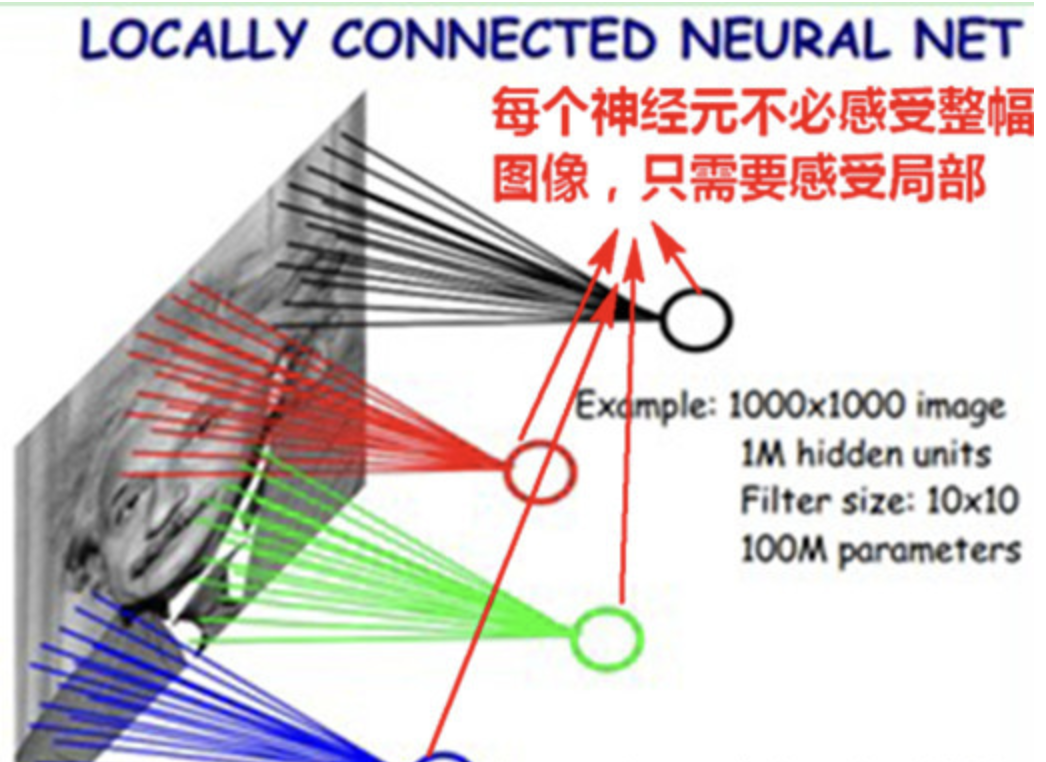黑色绿色红色蓝色这四个神经元分别链接了图示四个区域，而不是与图中单个像素相链接这就叫局部感受野。
权重共享
如果想要原始图像的一个特征图只需要一个卷积核即可，假设这个卷积核的是3 * 3大小的，那么要得到这个特征图只需要训练9个参数。也就是说整张图都是享用这个3 * 3的卷积核的参数这就是权重共享。如果想要更多的特征图只需要增加卷积核的个数即可。
池化下采样
池化有两种操作，最大池化和平均池化。假设池化的大小是2 * 2。最大池化直接以2 * 2大小的滑动窗口在输入的特征图上去最大值。平均池化同理但是取得是平均值。这样特征图变小可以减少训练的参数量。池化层中平均池化是有两个参数可以训练的，一个是偏置的值还一个是对平均结果乘一个系数值。最大池化没有参数（这里还不太确定，但应该是没有吧？？？但好像也可以有，比如加上一个偏置）。
结构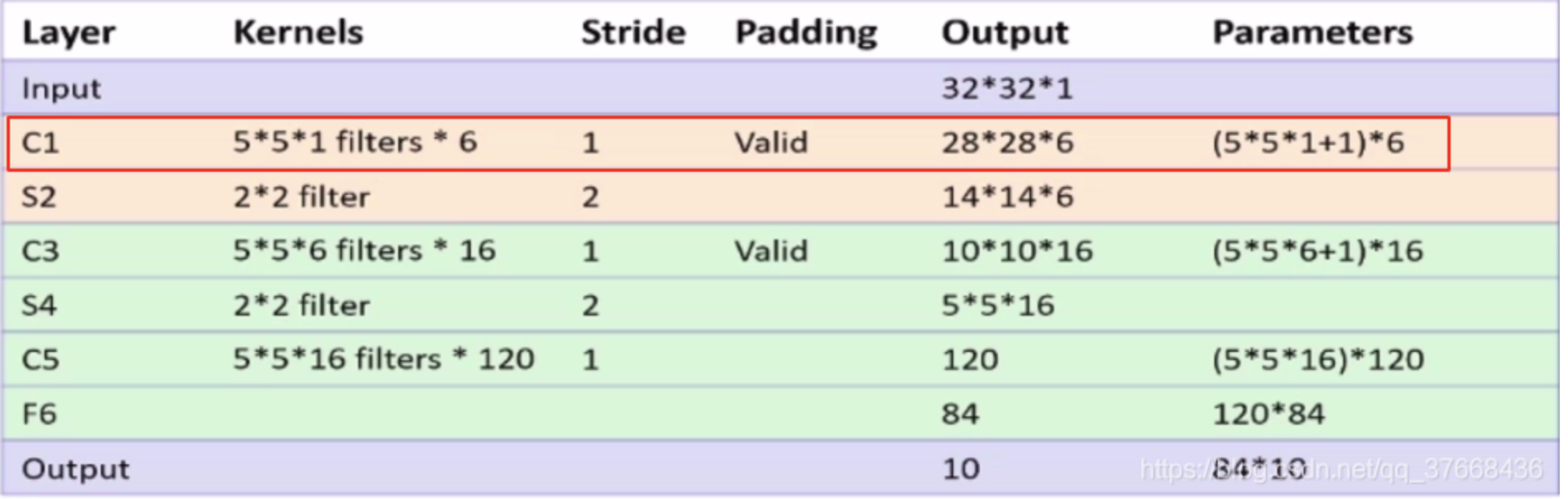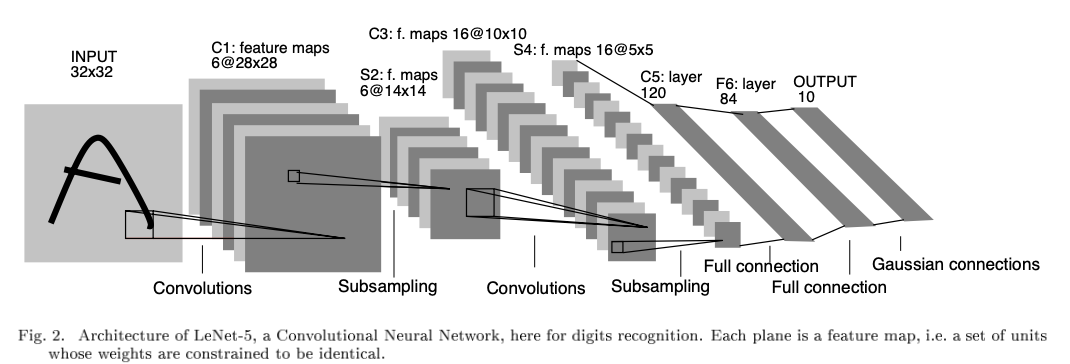代码
https://github.com/xmy0916/DLNetwork
网络结构部分：
class LeNet(nn.Module):
def __init__(self):
super(LeNet, self).__init__()
self.conv1 = nn.Conv2d(3, 6, 5)
self.relu = nn.ReLU()
self.maxpool1 = nn.MaxPool2d(2, 2)
self.conv2 = nn.Conv2d(6, 16, 5)
self.maxpool2 = nn.MaxPool2d(2, 2)

self.fc1 = nn.Linear(16 * 5 * 5, 120)
self.fc2 = nn.Linear(120, 84)
self.fc3 = nn.Linear(84, 10)

def forward(self, x):
x = self.conv1(x)
x = self.relu(x)
x = self.maxpool1(x)
x = self.conv2(x)
x = self.relu(x)
x = self.maxpool2(x)
x = x.view(-1, 16 * 5 * 5)
x = F.relu(self.fc1(x))
x = F.relu(self.fc2(x))
x = self.fc3(x)
return x

conv1特征图可视化：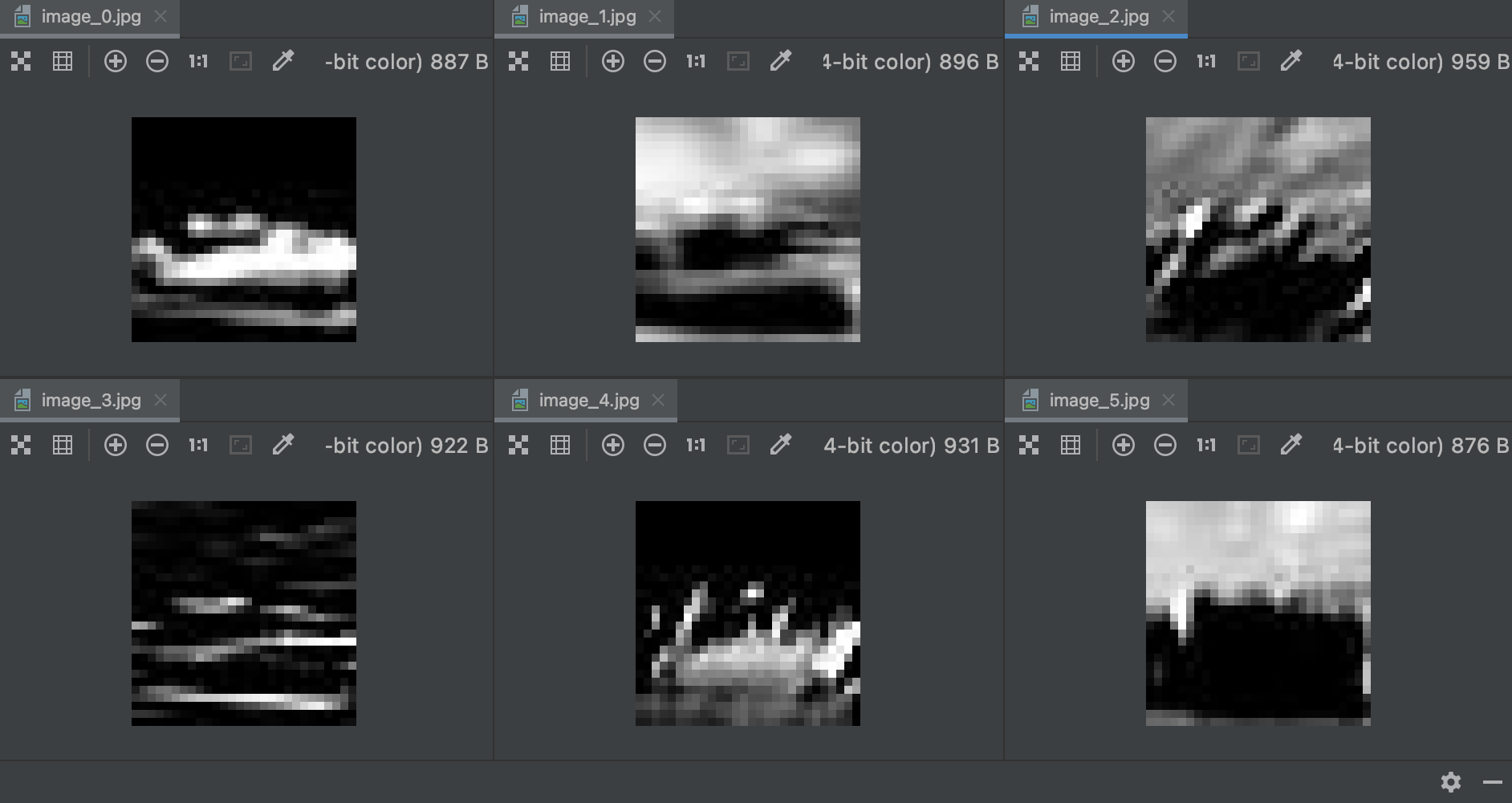展开全文深度学习 pytorch
• LeNet-5结构分析及caffe实现————卷积部分 原文地址：http://www.cnblogs.com/wangxiu/p/5513354.html 1、lenet-5的结构以及部分原理 2、caffe对于lenet-5的代码结构 图一 图一是整个LeNet-5的...
• 目录MINST数据集的介绍LeNet网络结构输入层C1层S2层C3层S4层C5层F6和输出层结果输出及损失函数的解释损失函数结果展示LeNet代码附详细注解 MINST数据集的介绍 MINST数据集是手写0到9组成的数据集，来自 250 个不同人...人工智能 python 卷积
• 二：基于tensorflow代码实现以及调参对比 首先实现一个lenet-5的代码 import tensorflow as tf import input_data mnist = input_data.read_data_sets('MNIST_data', one_hot=True) sess = tf....
• 2、caffe对于lenet-5的代码结构 图一 图一是整个LeNet-5的结构图，要点有：convolutions、subsampling、full connection、gaussian connection。 要点拆分： 1、convolution 是卷积操作，对应的概念有卷积...
• CNN网络架构演进：从LeNet到DenseNet 卷积神经网络可谓是现在深度学习领域中大红大紫的网络框架，尤其在计算机视觉领域更是一枝独秀。CNN从90年代的LeNet开始，21世纪初沉寂了10年，直到12年AlexNet开始又再焕发第...
• OpenCV基于LeNet-5和连接组件分析的数字识别的实例OpenCV基于LeNet-5和连接组件分析的数字识别的实例 OpenCV基于LeNet-5和连接组件分析的数字识别的实例 #include <opencv2/imgproc.hpp> #include <opencv2...
• ## LeNet

2020-06-02 10:13:20卷积神经网络
• 一、LeNet-5 简介 LeNet-5 是 Yann Lecun 于1998提出的神经网络架构，更是卷积神经网络的开山鼻祖，虽然该网络模型仅有 7 层神经网络结构，但在 MNIST 数据集上的识别精度高达 99.2%，是卷积神经网络首次在数字...
• 更新于：20180920 ...本文代码是theano、tensorflow 但实际完全可以用pytorch 来做啊。恩～～   这里学习了两个版本的代码，保存下，原理自己学： https://blog.csdn.net/hjimce/article/d...深度学习
• LeNet卷积模型实现Mnist手写体训练 摘要 LeNet卷积模型的模型 网络训练 预测正确率和分析 -1. Mnist数据集的识别结果 -2. 外部数据的识别结果及分析 -3. 总结 代码 LeNet卷积模型的模型： LeNet卷积模型共七层。 ...
• ## LeNet-5模型实现

千次阅读 2018-07-22 18:22:56
现在逐渐的开始接触深度学习的知识，最近看了Ng老师的深度学习视频，看到里面介绍了几种经典的卷积神经网络其中就包含LeNet-5所以就照葫芦画瓢，用tensorflow实现了一下最原始的LeNet-5模型，顺便也是为了学习...Matlab
• LeNet以及ResNet实现 LeNet 基本结构 代码部分 导入包 构建不同的层 forward ResNet 基本结构 代码部分 导入包 ResBlock ResNet18（与原论文稍有变化） LeNet 基本结构 输入图片大小为[3, 32, 32] C1: (kernel_size=...卷积神经网络 深度学习
• 此例程出自《TensorFlow实战Google深度学习框架》6.4.1小节 经典卷积网络模型之LeNet-5模型，具体可搜索“LeNet-5, convolutional neural networks”。 例程中的网络模型与原始LeNet-5模型不太一样，网络结构如下：...
• 卷积层的常用实现方式就是利用这一点，将卷积层的前向传播变成一个巨大的矩阵乘法： 1. 输入图像的局部区域被im2col操作拉伸为列。比如，如果输入是[227x227x3]，要与尺寸为11x11x3的滤波器以步长为4进行卷积，就...深度学习 神经网络
• 文章目录前言1、卷积神经网络的基本步骤1、卷积神经网络计算convolution2、感受野以及卷积核的选取3、全零填充...分析2、经典卷积网络讲解1、LeNet2、AlexNet3、VGGNet4、InceptionNet5、ResNet6、经典卷积网络总结总结...卷积 神经网络 深度学习 网络 tensorflow
• LeNet5网络既经典又重要，网上关于该模型的解释数不胜数，我也就不做重复的劳动了，直接给出个人认为比较好的介绍的链接，只对其中部分内容作简要介绍，算是自己的笔记，给自己看的。 论文地址：...深度学习 卷积 pytorch卷积 神经网络 深度学习 卷积神经网络 pytorch
• 文章目录OCR1.1实现简单lenet5分类概述代码分析数据读取部分：网络训练部分运行效果完整代码ps：欢迎留言指正 概述 上篇1.0基本介绍了数据集的预处理，这次接着写入门的识别部分（tensorflow(cpu)) 原始数据集是有五...深度学习 tensorflow 人工智能 自然语言处理 算法
• 1. 介绍LeNet-5​网络结构 2. 利用TensorFlow实现该网络结构，并测试识别mnist手写数据集深度学习 tensorflow 人工智能 卷积神经网络
• 深度学习-卷积神经网络-实例及代码1（入门）—利用Tensorflow和mnist数据集训练单层前馈神经网络/感知机实现手写数字识别 深度学习-卷积神经网络-实例及代码2（初级）—利用Tensorflow和mnist数据集训练简单的深度...计算机视觉 tensorflow python 卷积神经网络 深度学习
• 阅读LeNet论文 LeNet原论文地址 原论文共分了11个部分，我们所听说的LeNet-5是在Section II第二部分讲解的，其他部分可以自行阅读论文。 不算输入层，LeNet-5一共有7层网络
• 当输入图片为28*28*1的图片时，为适应图片大小修改网络如下： 正向传播代码实现： 反向传播代码实现： 在测试时，也要对x进行调整 ...
• 进入正文一、LeNet网络简介LeNet网络简介LeNet网络的背景1.1闪光点：定义了CNN的基本组件，是CNN的鼻祖。LeNet是卷积神经网络的祖师爷LeCun在19...
• 基于LeNet的TensorFlow实现2.1 程序代码分析2.2 实验演示3. 结尾参考资料 1. 目标与背景 在这一讲中，我们将讲解深度学习的编程工具Tensorflow的基础使用规则。最初的深度学习编程工具主要由研究人员义务开发，免费...卷积 神经网络 tensorflow 深度学习
• ## LeNet5

2021-07-05 08:21:04
文章目录一、简介二、网络结构三、代码实现 TODO参考 一、简介 LeNet是LeCun在1998年提出，用于解决手写数字识别的视觉任务。一般认为，卷积神经网络是从这里开始的。LeNet5一共有七层。两个卷积，两个池化，三个全...神经网络...